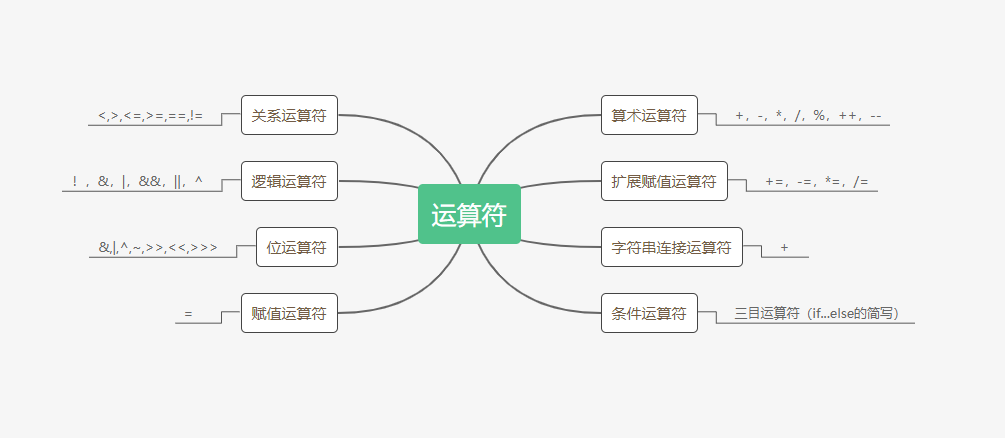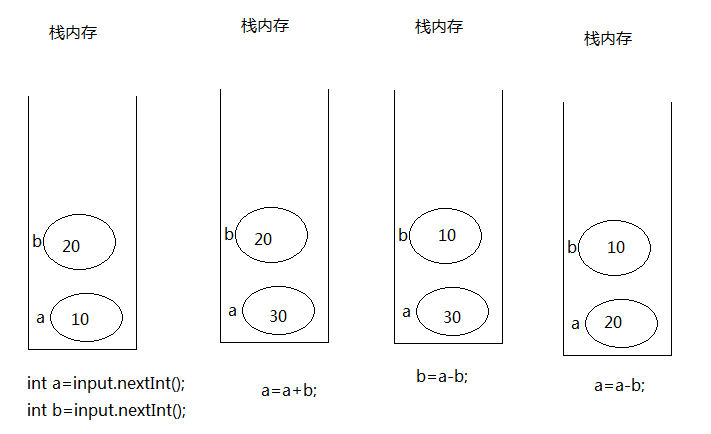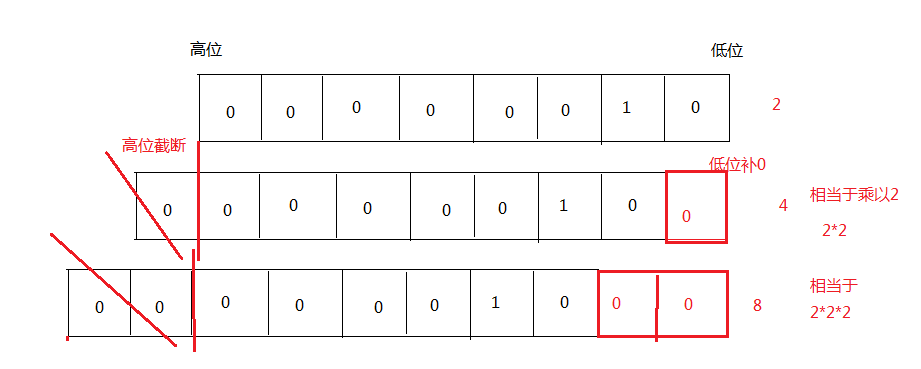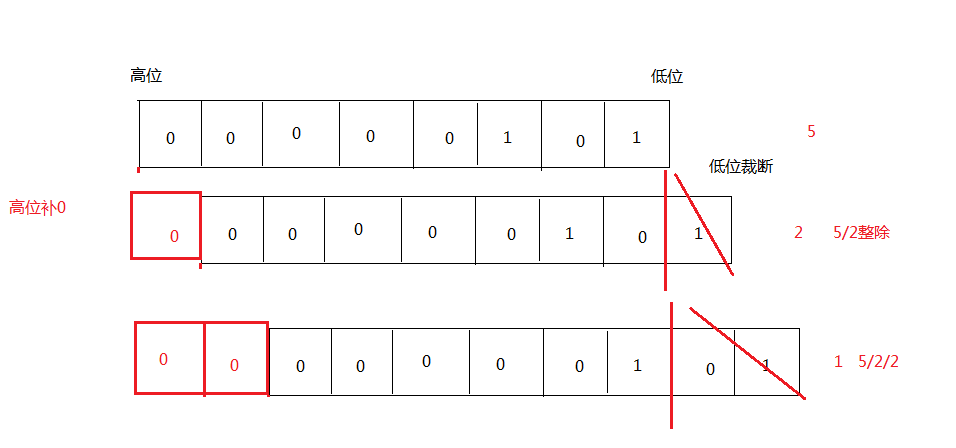【Java】运算符学习笔记

Java语言支持的运算符分别介绍

1、算术运算符

public class Test {
public static void main(String [] args) {
int a = 10,b = 20;  //一次性声明两个int类型的变量
System.out.println(a + b);
System.out.println(a++ + b);//30,因为++在a之后，所以先秇行a+b，将结果输出，这个时候a的值为11
System.out.println(a+ b++);//31 ,b的值21
System.out.println(a+ ++b);//33  ,11+22
System.out.println(a++ +b);  //执行的是a++
System.out.println(a+ "\t" +b);

/**
a++;或者++a ;没区别
在执行运算时或者输出时，才有区别
以下两句代码，有区别  一句代码，执行两个操作，自加和输出，就需要分先后
System.out.println(a++);
System.out.println(++a);
*/

}
}


2、赋值运算符

import java.util.Scanner;
public class Test {
public static void main(String [] args) {
Scanner input= new Scanner(System.in);
System.out.println("请输入第一个数:");
int a = input.nextInt();
System.out.println("请输入第二个数:");
int b = input.nextInt();
System.out.println("交换之前:a="+a+"\tb="+b);

/**不使用第三变量交换两个变量值*/
a = a + b;//将a+b的结果赋值到a中存储
b = a - b;//将a-b的结果赋值到b中存储
a = a - b;//将a-b的结果赋值 到a中存储
System.out.println("交换之后:a= "+ a +"\tb=" + b);

}
}3、扩展运算符

举例:
byte b1 = 10;
b1 += 1;
// 编译器编译成
b1 = (byte)(b1 + 1);


4、关系运算符

public class Test {
public static void main(String [] args) {
boolean b = 5 != 8;  //语句  ,b的值是在编译期间计算完成 ,因为"="右侧没有变量
System.out.println(b);  //true
/**关系运算符  >,<,>=,<=,==,!=*/
/**赋值运算符  =

关系运算符的优先级 大于赋值运算符   ,先计算关系，然后再赋值
5 != 8 结果为true,
boolean b = true;

*/
}
}


“==”只能比较基本数据类型的值是否相等，

float与double用于科学计算或工程计算
BigDecimal 精确的浮点型，用于商业领域

5、位运算符
1）<< :左移位 ，向左移动一位，相当于乘以2

public class Test {
public static void main(String [] args) {
int a = 2;
int c = a << 1;  //向左移动1位  ,相当于乘以2
System.out.println("c=" + c);
int d = a << 2;  //向左移动2位  ,相当于乘以4
System.out.println("d=" + d);

}
}2）

右移位:   向右移动1位，相当于除以2
>>  :右移
>>> ：无符号右移

public class Test {
public static void main(String [] args){
int a = 5;
int c= a >>> 1;  //向右移动1位  ,相当于除以2
System.out.println("c=" + c);
int d = a >>> 2;  //向右移动2位  ,相当于除以4
System.out.println("d=" + d);

}
}6、条件运算符

int score = 80; int x = -100;
string type = score < 60 ? "不及格" : "及格";
int flag = x > 0 ? 1 : (x == 0 ? 0 : -1);
system.out.println("type = " + type);
system.out.println("flag = " + flag);

//结果为👇
type = 及格
flag = -1


7、字符串连接运算符
1）“+” 除用于算术加法运算外，还可用于对字符串进行连接操作

int id = 800 + 90;
String s = "hello" + "world";


2）“+”运算符两侧的操作数种只要有一个是字符串（string）类型，系统给会自动将另一个操作数转换为字符串然后再进行连接。

int c = 12;
System.out.println("c=" + c);


3）当进行打印时，无论任何类型，都自动转为字符串进行打印

8、逻辑运算符
1）逻辑与 和 短路与的区别

&&  : 左右只能是boolean操作数
左侧为false,右侧不计算  (提高效果)
&  : 左右可以是boolean操作数， 作用：逻辑与
也可以是数值型                   ,  作用 :按位与
左侧为false,依然计算右侧表达式

public class Test {
public static void main (String [] args) {
/**知路与(并且)&&  逻辑与  &*/
boolean b = 8 < 5 && 5 / 0 > 2;
System.out.println (b); //false    8<5的结果为false   5/0>2没有计算

System.out.println (8 & 4);  //0  二进制的按位与   , 同为1时结果为1
/**
1000       -->8的二进制
0100       -->4的二进制
------------------
0000    同为1时则为1    --》计算结果为0
*/
//System.out.println (8 && 4);操作数有误，因为&&的左右必须是boolean型

boolean b1 = 8 < 5 & 5 / 0 > 2;
System.out.println (b1);  //by zero ,ArithmeticException算术异常
//运行时异常（运行时发生了不正常的情况）
//8<5的结果为false,依然计算&右侧表达式

/**
&&  :左侧表达式的结果为false，肯本就不计算右侧表达式  (开发时使用)
&   :无论左侧表达式是否为false,依然计算右侧表达式  (面试，考试时)
*/
}
}


(2)逻辑或 和短路或的区别

||  : 左右只能是boolean操作数
左侧为true,右侧不计算  (提高效果)
| : 左右可以是boolean操作数， 作用：逻辑或
也可以是数值型                   ,  作用 :按位或
左侧为true,依然计算右侧表达式

public class Test {
public static void main (String [] args) {
/**短路或(或者)||  逻辑或  ｜*/
boolean b = 8 > 5 || 5 / 0 > 2;
System.out.println (b);  //true,  左侧表达式的结果为true,不计算右侧

System.out.println (8 | 4);  //按位或  ，有一个为1，结果为1
/**
1000 -->8的二进制
0100 -->4的二进制
-------------
1100  -->12
*/

boolean b1 = 8 > 5 | 5 / 0 > 2;
System.out.println (b1);  //by zero,左侧表达式的结果为true,依然计算右侧表达式

}
}